Function Repository Resource:

# DiscreteHartleyTransform

Compute the discrete Hartley transform of a list

Contributed by: Jan Mangaldan
 ResourceFunction["DiscreteHartleyTransform"][list] computes the discrete Hartley transform of a list of complex numbers.

## Details

The discrete Hartley transform vs of a list ur of length n is defined to be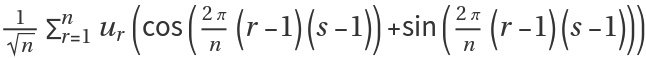. The normalization is chosen such that the transform is its own inverse.
The discrete Hartley transform is sometimes defined to have the normalization constant 1 or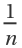instead.
The discrete Hartley transform is real-valued for real-valued data.
The list of data supplied to ResourceFunction["DiscreteHartleyTransform"] need not have a length equal to a power of two.
The list given in ResourceFunction["DiscreteHartleyTransform"][list] can be nested to represent an array of data in any number of dimensions.
The array of data must be rectangular.
If the elements of list are exact numbers, ResourceFunction["DiscreteHartleyTransform"] begins by applying N to them.
ResourceFunction["DiscreteHartleyTransform"] can be used on SparseArray objects.

## Examples

### Basic Examples (1)

Compute a discrete Hartley transform:

 In:=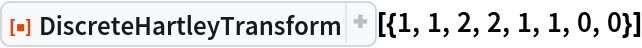Out=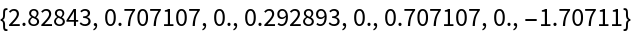### Scope (4)

x is a list of real values:

 In:=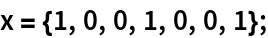Compute the Hartley transform with machine arithmetic:

 In:=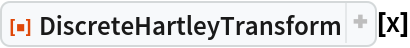Out=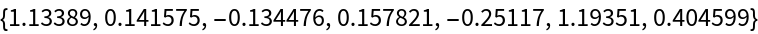Compute using 24-digit precision arithmetic:

 In:=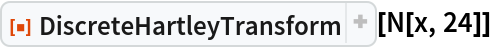Out=Compute a complex Hartley transform:

 In:=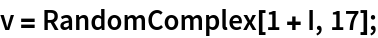In:=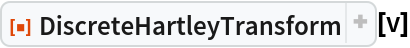Out=This is equivalent to separately taking the Hartley transforms of the real and imaginary parts:

 In:=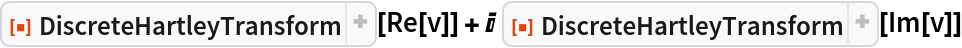Out=Compute a 2D Hartley transform:

 In:=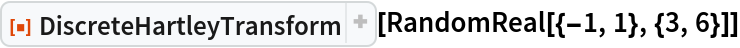Out=x is a rank 3 tensor with nonzero diagonal:

 In:=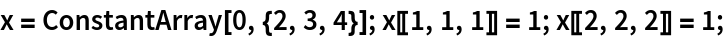Compute the 3D Hartley transform:

 In:=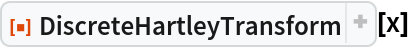Out=### Applications (2)

Use the discrete Hartley transform to compute the power spectrum of "white noise":

 In:=Out=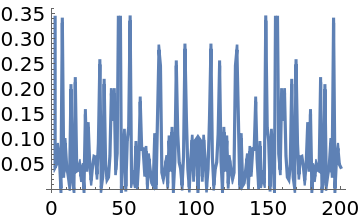Show the logarithmic spectrum, including the first (DC) component:

 In:=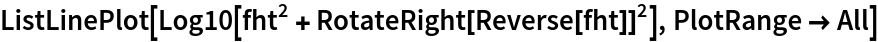Out=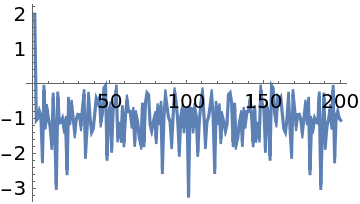Compute discrete cyclic convolutions to smooth a discontinuous function with a Gaussian:

 In:=In:=Out=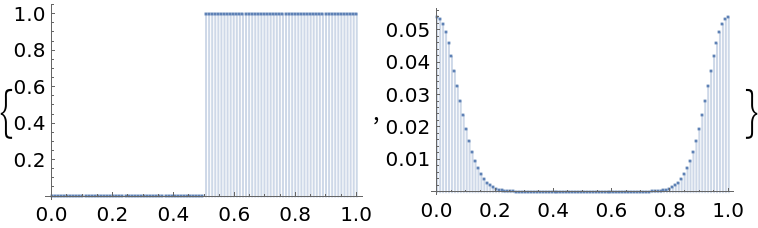Compute the cyclic convolution:

 In:=Show the original and smoothed function:

 In:=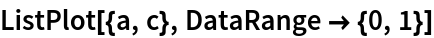Out=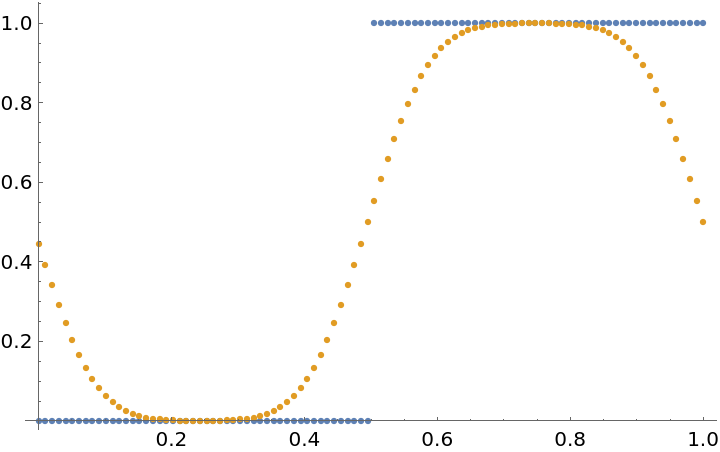The convolution is consistent with ListConvolve:

 In:=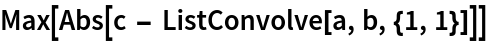Out=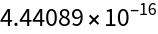### Properties and Relations (4)

Compute the discrete Hartley transform from its definition:

 In:=In:=Out=DiscreteHartleyTransform is faster:

 In:=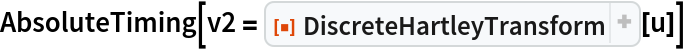Out=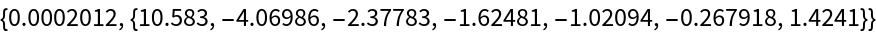In:=Out=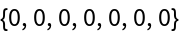Compute the discrete Hartley transform of a vector by multiplying it with the Hartley matrix:

 In:=In:=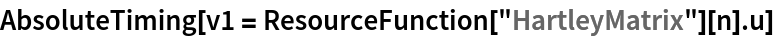Out=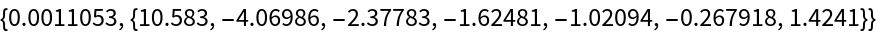DiscreteHartleyTransform is faster:

 In:=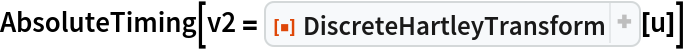Out=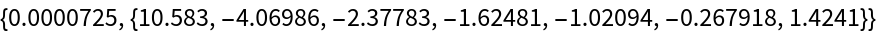In:=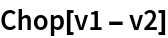Out=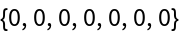The discrete Hartley transform is its own inverse:

 In:=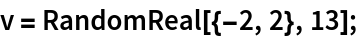In:=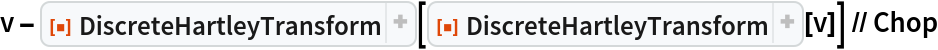Out=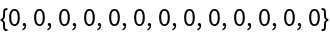A list of numbers:

 In:=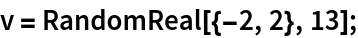Compute its discrete Hartley transform:

 In:=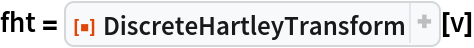Out=Use the discrete Hartley transform to compute the discrete Fourier transform:

 In:=Out=Compare with the result of Fourier:

 In:=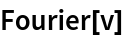Out=## Version History

• 1.1.0 – 13 March 2023
• 1.0.0 – 13 February 2023